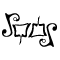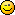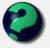# Math Is Fun Forum

Discussion about math, puzzles, games and fun.   Useful symbols: ÷ × ½ √ ∞ ≠ ≤ ≥ ≈ ⇒ ± ∈ Δ θ ∴ ∑ ∫  π  -¹ ² ³ °

You are not logged in.

## #1 2005-10-23 17:33:30

ryos
MemberRegistered: 2005-08-04
Posts: 394

### Monotonicity

Discuss the monotonicity of f(x) = 30x^9 + 2x^8 + 300x^7 - (17/2)x^6 - x^5 + 3x^4 +973x^3 - 200x^2 + x + 1 .

Answer: We will expand the polynomial into longhand form:
30xxxxxxxxx+2xxxxxxxx+300xxxxxxx-(17/2)xxxxxx-xxxxx+3xxxx+973xxx-200xx+x+1.

As you can see, f(x) is highly monotonous. Even the best of orators would be hard pressed to read it without slipping into a monotone. Note also that the monotonicity of f decreases as it goes along; the monotonous x's are broken up more frequently by other stuff, making it far more...polytonous?

El que pega primero pega dos veces.

Offline

## #2 2005-10-23 19:53:41

MathsIsFunRegistered: 2005-01-21
Posts: 7,710

### Re: Monotonicity

Yep, that "x" sure is monotonousBut a more formal definition says it is monotonic if it is either always increasing in x (or always decreasing in x), in other words always a general up-slope (or always a general down-slope).

So monotonic can't have humps and bumpsExample: y = 2x

(I think FLAT also qualifies, so y=2 would work, and they say "strict" if it doesn't qualify)

Is your polynomial monotonic? Plot it in Excel and see. (Also you may be able to do a derivative and find if the slope ever changes sign ... I think that might work, but better read up on it first!)

"The physicists defer only to mathematicians, and the mathematicians defer only to God ..."  - Leon M. Lederman

Offline

## #3 2005-10-24 02:18:13

mathsyperson
ModeratorRegistered: 2005-06-22
Posts: 4,900

### Re: Monotonicity

What about something like y = x³? That's mostly always increasing, but it also has a flat bit.

Why did the vector cross the road?
It wanted to be normal.

Offline

## #4 2005-10-24 03:33:08

ryos
MemberRegistered: 2005-08-04
Posts: 394

### Re: Monotonicity

Plot of f(x) = 30x^9 + 2x^8 + 300x^7 - (17/2)x^6 - x^5 + 3x^4 +973x^3 - 200x^2 + x + 1

Note the scale on the axes.

Last edited by ryos (2005-10-24 03:33:50)

El que pega primero pega dos veces.

Offline

## #5 2005-10-24 08:57:17

MathsIsFunRegistered: 2005-01-21
Posts: 7,710

### Re: Monotonicity

Cool graph .. I thought it would go down for a bit because of the negative terms.

So not monotonic.

Ummm ... if you have to say more, you could say it IS monotonic for negative x. You could also do the derivative and find the turning points.

f(x) = 30x^9 + 2x^8 + 300x^7 - (17/2)x^6 - x^5 + 3x^4 +973x^3 - 200x^2 + x + 1
f'(x) = 270x^8 + 16x^7 + 2100x^6 - 51x^5 - 5x^4 + 12x^3 +2919x^2 - 400x + 1

Now solve for f'(x)=0, which I can't do because it has way too many terms, so that was fairly useless."The physicists defer only to mathematicians, and the mathematicians defer only to God ..."  - Leon M. Lederman

Offline

## #6 2005-10-27 17:33:52

ryos
MemberRegistered: 2005-08-04
Posts: 394

### Re: Monotonicity

I should have known better than to make fun of Math in a Math forum.

El que pega primero pega dos veces.

Offline